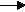gcsescience.com                                                 18                                                 gcsescience.com

Atomic Structure

What do the Symbols and Numbers Mean
in
Chemical Equations?

When we are balancing chemical equations
we need to know what the symbols and numbers mean.

When we write an equation for a chemical reaction,
for example       Mg  +  Cl2MgCl2
each atom or ion of an element
is represented by a chemical symbol.

Mg is the chemical symbol for magnesium.
Mg
means one magnesium atom (Mg = Mg1 see below).
Cl means one chlorine atom. Cl2 means two chlorine atoms.

The formula of a compound cannot be changed.
The formula is shown by small numbers written after
(and a little below) each element in the compound.

KCl  is an ionic compound.
KCl  means one potassium ion with one chlorine ion
(the number one is never written, KCl  = K1
Cl1).
Na
2 means two sodium ions with one oxygen ion.
Al
2O3 means two aluminium ions with three oxygen ions.

We shall look at two examples of unbalanced equations.
You can balance any chemical equation by applying the rules
shown in these examples. Just click on an example below.

Example 1.   The reaction of potassium with chlorine.

Example 2.       The reaction of lithium with oxygen.

gcsescience.com   The Periodic Table   Index   Balancing Equations Quiz   gcsescience.com